# Stabilization of Adjoint Sensitivities in Chaotic Flows

Suneth Warnakulasuriya did his honours’ project at the Chair of Structural Analysis (TUM) and
was supervised by Michael Andre M.Sc., Altug Emiroglu M.Sc., PD Dr.-Ing. habil. Roland Wüchner and Prof. Dr.-Ing. Kai-Uwe Bletzinger.

With the rise of the computational power at dispense, the computation of numerically complex problems have become increasingly popular expanding to new branches. One of which is Optimization. Optimization, a field which is known to have started with Euclid, now has merged with many engineering fields as a key component in product design. This is true for Computational Fluid Dynamics (CFD) and Computational Solid Mechanics (CSM) as well. As the urge for cost reduction and higher performance kept on rising; the demand for optimization grew at a rapid pace. Optimization of a design can be carried out using gradient based methods where sensitivities and objective values are used or non-gradient based methods where only objective values are being used. Sensitivities are computed mainly in two different methods in gradient based methods. First one being the finite difference method, where one variable is perturbed while all the other design variables are kept constant. This method involves high computational effort if number of design variables are high because, it involves O(n + 1) complexity (i.e. n + 1 simulations) where n is the number of design variables. The second method is to use adjoint sensitivities. This method only require two simulations irrespective of number of design variables for a given objective.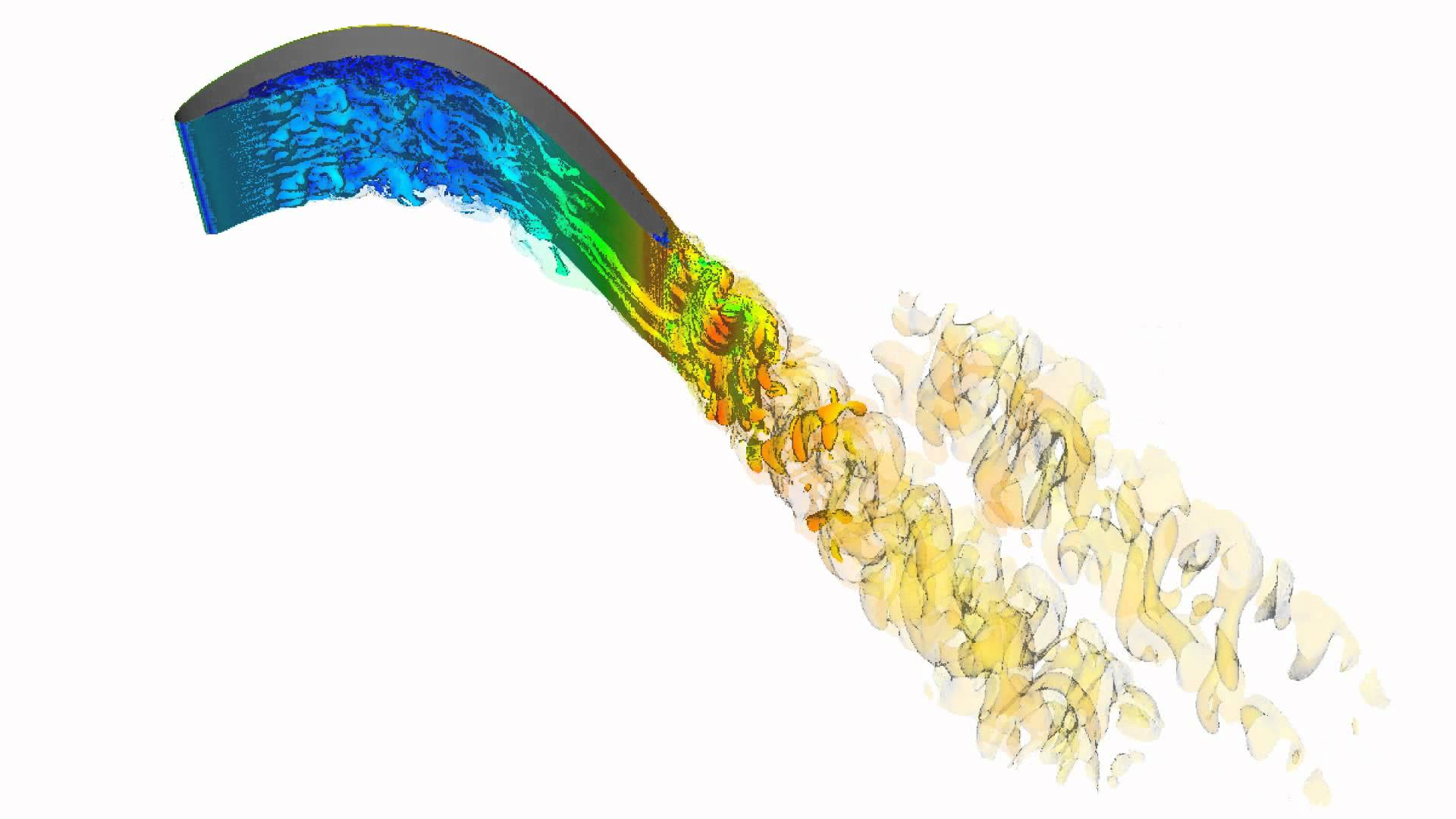Fig.1: Chaotic flow over a turbine blade

High-fidelity simulations like Large Eddy Simulations (LES) are essential for accurately simulating turbulent chaotic flows. If sensitivities are required for problem which needs to be simulated using LES, then it requires transient adjoint system of equations to be solved because, finite difference method for a simulation with LES will be im-practical due to high computational cost arising because of high number of design variables (i.e. node coordinates in the mesh). Therefore, adjoint sensitivities became more popular.

This project focuses on theoretical basis of transient adjoint sensitivities and its inherent problem in chaotic flows and proposes few different methods to overcome it which is implemented in open source Kratos FEM framework. A pilot project is introduced to validate the introduced methods to overcome this issue (refer Figure 2).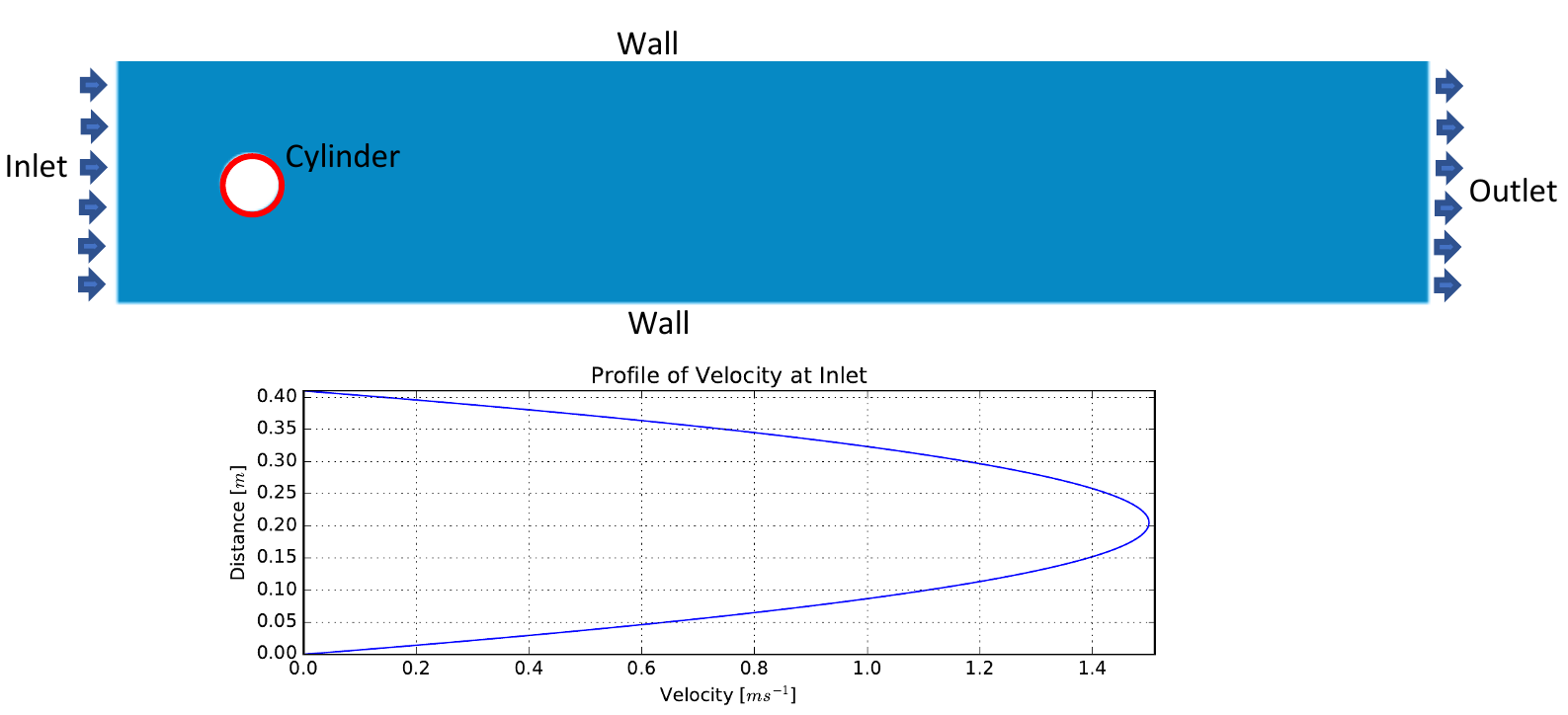Fig.2: Pilot project configuration

The adjoint variables grow exponentially backward in time in transient chaotic flow which make derivatives derived from adjoints useless. Chaos theory also provides evidence that solutions of non-linear Partial Differential Equation (PDE) are sensitive to perturbations in initial conditions or parameters . The solutions for Navier-Stokes equations also exhibit the similar behaviour which results with diverging adjoint variables in a chaotic flow simulation which is illustrated in Figure 3.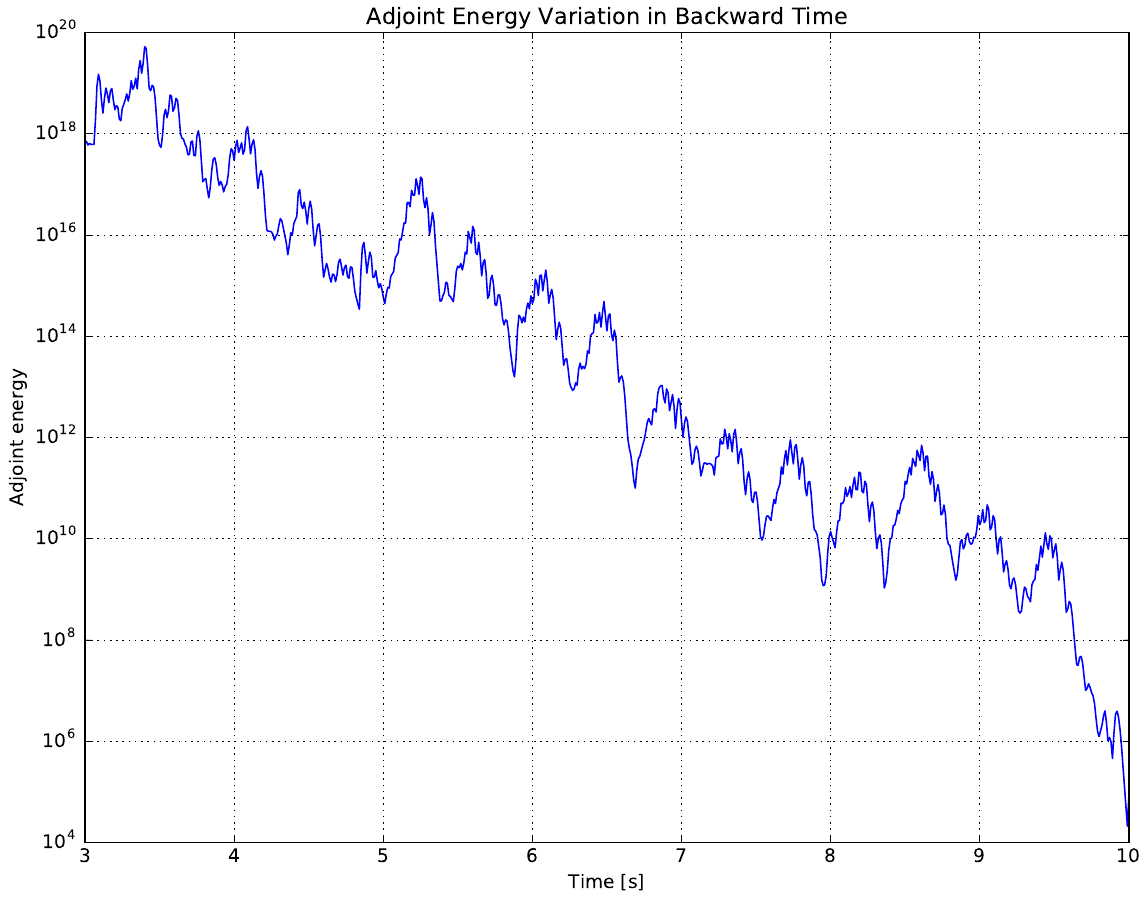Fig.3: Adjoint energy variation in backward time

In order to stabilize the growing energy in adjoint fields, energy needs to be taken out from the adjoint system. Therefore numerical diffusion was added to elements which contribute towards the exponential growth of the adjoint energy. Two different numerical diffusion addition methods were studied namely Eigen method and singular value method. β is the controlling parameter introduced in the numerical diffusion addition methods (i.e. β = 0 then no numerical diffusion added to the system). Figure 4 illustrates adjoint energy variation after appying numerical diffusion based on the Eigen method. Figure 5 illustrates adjoint energy variation after applying numerical diffusion based on singular value method.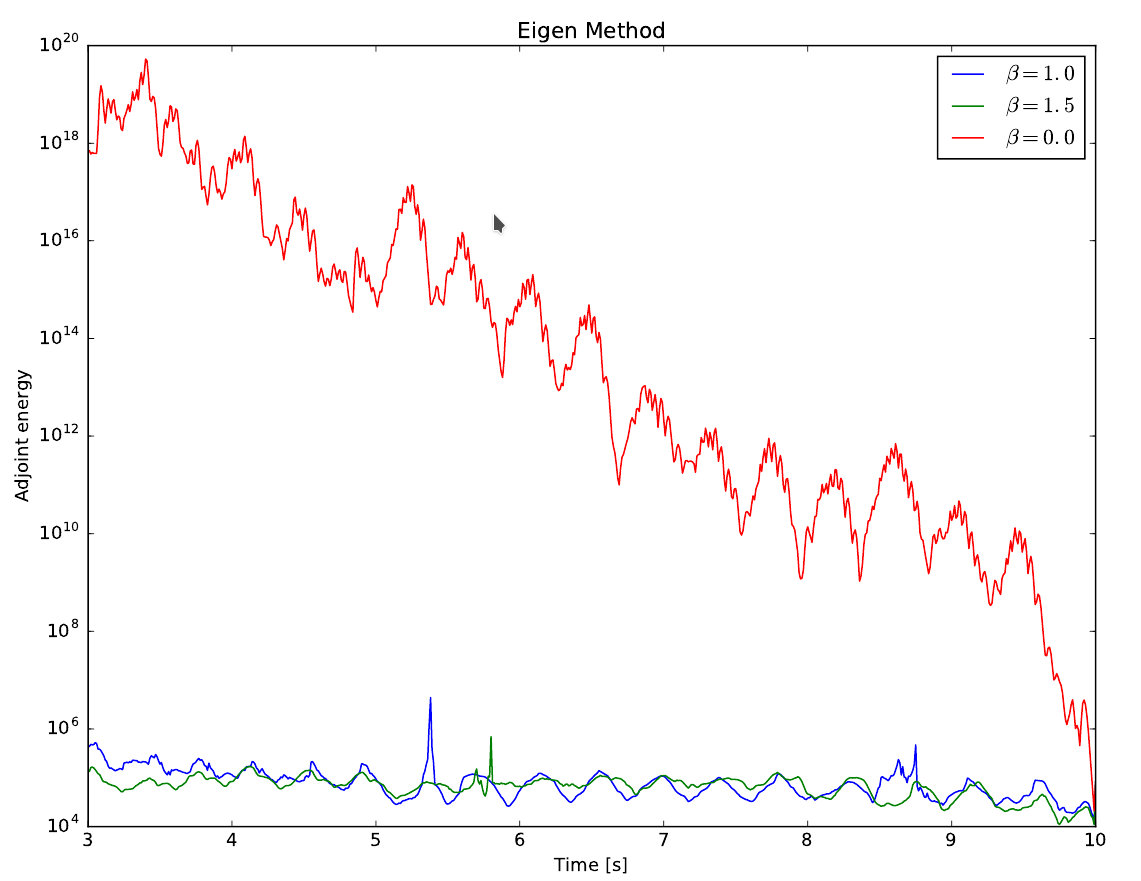Fig.4: Adjoint energy variation in eigen method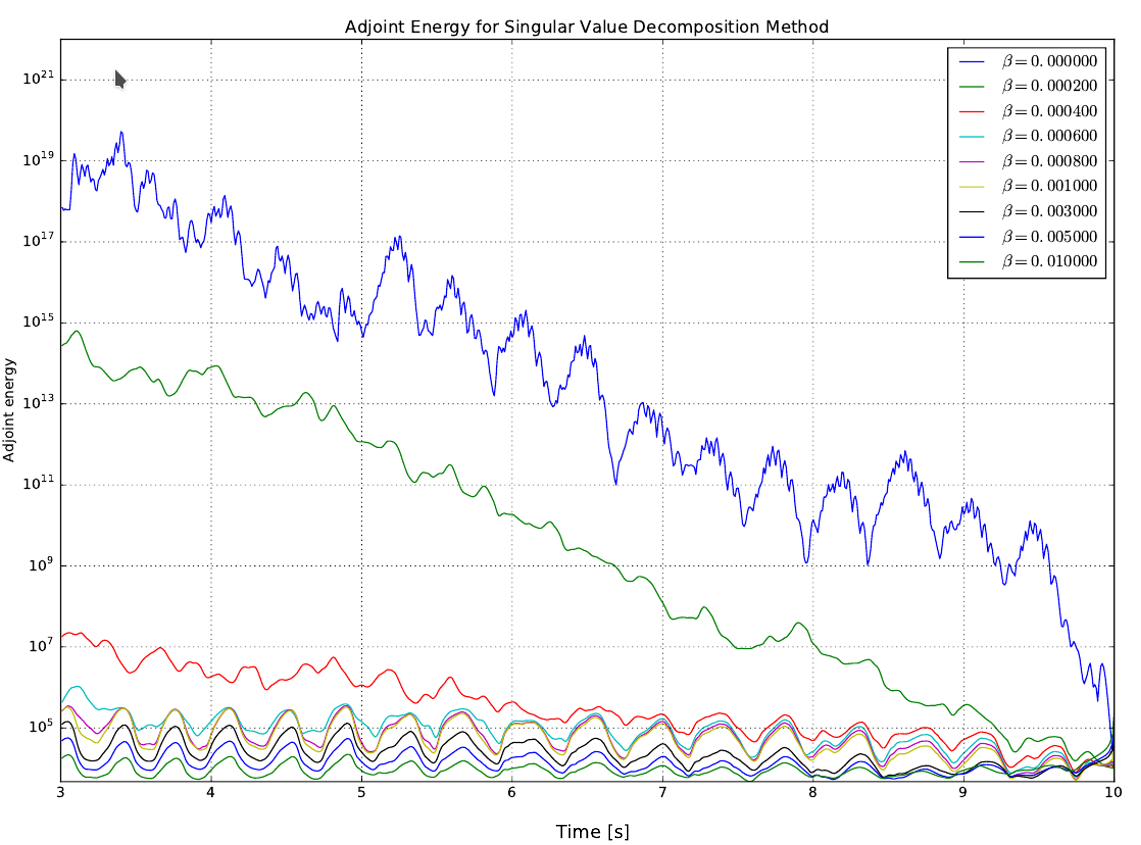Fig.5: Adjoint energy variation in singular value method

Validation of computed stabilized adjoint sensitivites were carried out in comparison with the finite differencing radial sensitivities for the pilot project for different values of β. Figure 6 illustrates validation results of the singular value method.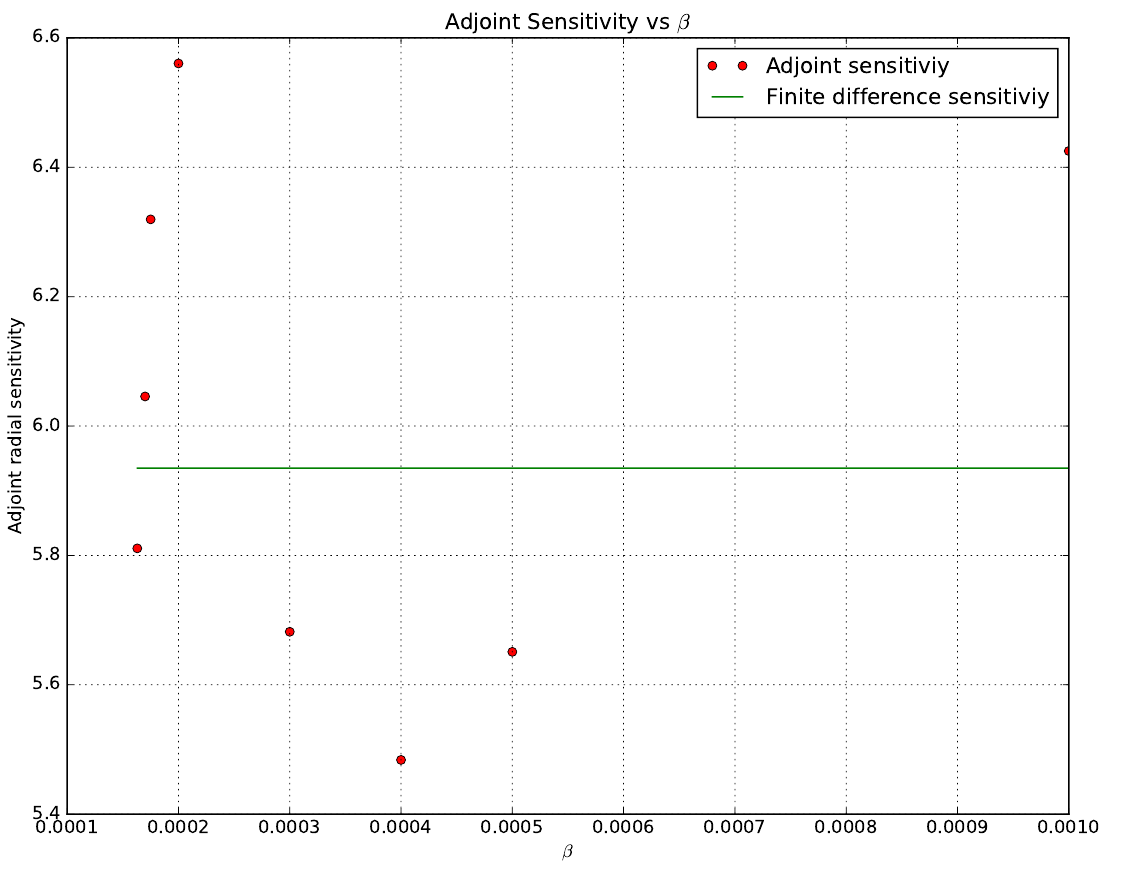Fig.6:Validation of singular value method

Two different methods of added numerical diffusion were identified to stabilize exponentially growing adjoint fields namely, Eigen method and singular value method. All of these methods were successfull in stabilizing the adjoint fields, but the Eigen method has proven to be more sensitive towards the magnitude of added numerical diffusion, even the direction of the sensitivities changes with β value. Singular value method without pressure coupling exhibited quick convergence behaviour, whereas same method with pressure coupling slow convergence. The accuracy of the singular value method with pressure coupling was higher than in the same method without the pressure coupling.

#### References

  Wang, Q. and Gao., J. The drag-adjoint field of a circular cylinder wake at reynolds numbers 20, 100 and 500. Journal of Fluid Mechanics, 730 pp. 145-161, 2013.  Blonigan, P. J. and Chen, R. and Wang, Q. and Larsson, J. Towards adjoint sensitivity analysis of statistics in turbulent flow simulation Proceedings of the Summer Program, pp. 229, 2012.  Aceves, A. and Adachihara, H. and Jones, C. and Lerman, J. C. and McLaughlin, D. W. and Moloney, J. V. and Newell, A. C. Chaos and coherent structures in partial differential equations Physica D: Nonlinear Phenomena 18 (1), pp. 85-112, 1986.  Wilcox, D. C. and others Turbulence modeling for CFD DCW industries La Canada, CA 1998.